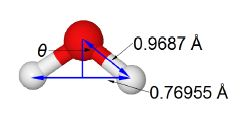# Molecular Structure: Theoretical Explanation

The water molecule has an O—H bond distances of 0.9687 x 10-10 m and the distance between the two hydrogen atoms is 1.5391 x 10-10 m. Calculate the H—O—H bond angle.Water O = red, H = gray

The water molecule forms an equilateral triangular structure. We can then bisect the H-0-H angle to make two right angled triangles, the right hand triangle is drawn in below Figure. As this is now a right angled triangle we can use trigonometric functions where θ is the half angle for H-O-H. The distance multiple of 10-10 m is called an angstrom with the symbol A, it is a common and convenient unit used by Chemists as it is of the order of bond distances and atomic radii.But as this is only half of the water bond angle, the bond angle is

H – O – H = 205.20